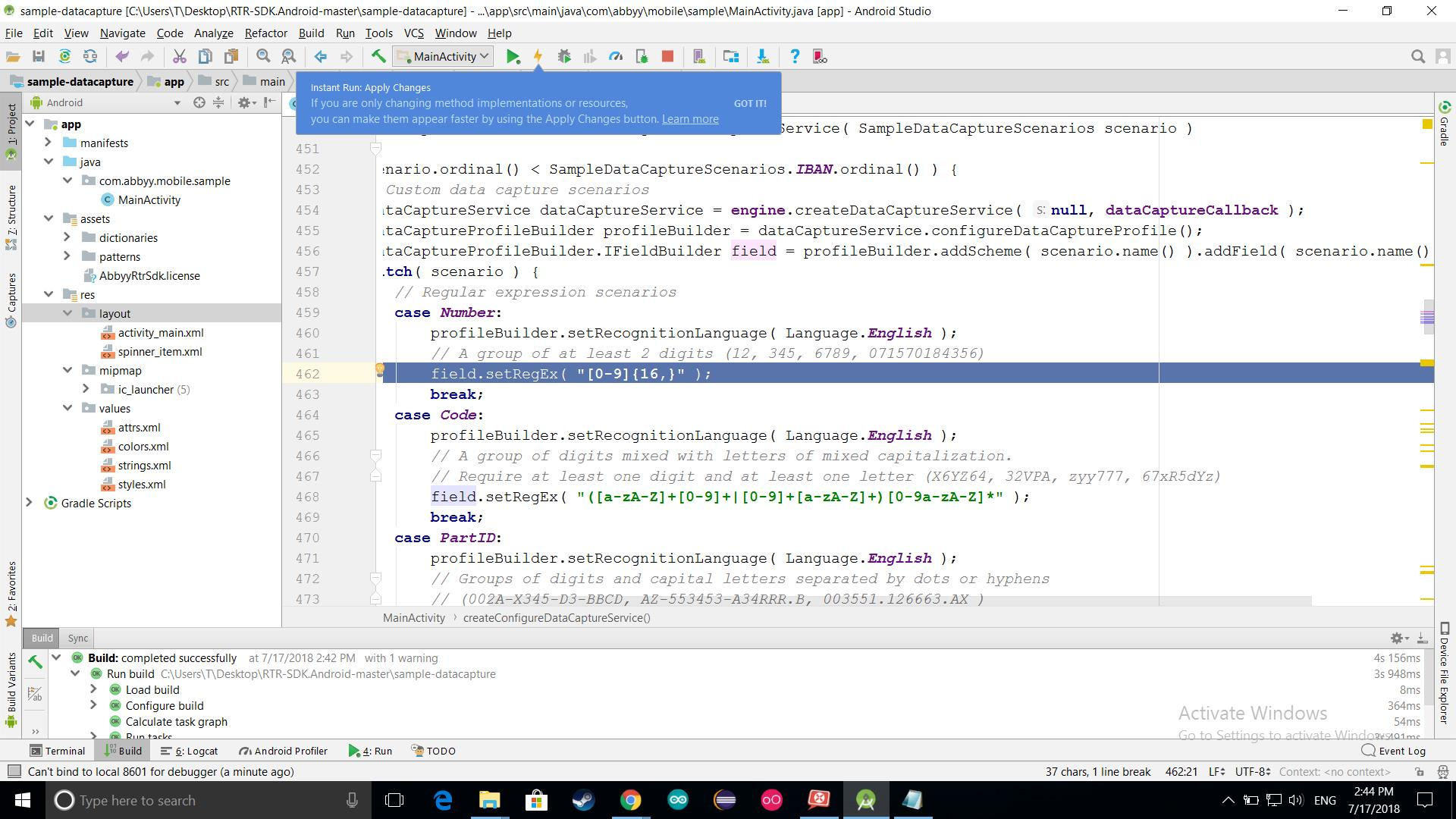# Community

## number scanner settingshi

here the app looks for a 16-digits number , but what i need is to scan for a number like this :

"1234 5678 9123 4567"

i mean 4 part of 4-digit numbers ; so what do i have to write in this line of the code.

please answer me .i have to complate my app in these three days

0 out of 0 found this helpful

•Hi

You could try something like

^(\d{4}\s\d{4}\s\d{4}\s\d{4})

As said by https://regex101.com/

/ ^(\d{4}\s\d{4}\s\d{4}\s\d{4}) / gm
^ asserts position at start of a line 1st Capturing Group (\d{4}\s\d{4}\s\d{4}\s\d{4}) \d{4} matches a digit (equal to [0-9]) {4} Quantifier — Matches exactly 4 times \s matches any whitespace character (equal to [\r\n\t\f\v ]) \d{4} matches a digit (equal to [0-9]) {4} Quantifier — Matches exactly 4 times \s matches any whitespace character (equal to [\r\n\t\f\v ]) \d{4} matches a digit (equal to [0-9]) {4} Quantifier — Matches exactly 4 times \s matches any whitespace character (equal to [\r\n\t\f\v ]) \d{4} matches a digit (equal to [0-9]) Global pattern flags
g modifier: global. All matches (don't return after first match) m modifier: multi line. Causes ^ and \$ to match the begin/end of each line (not only begin/end of string)
•Hi,

Please also find the information about all supported syntax in the Regular Expressions section of the RTR SDK documentation.

•i found this :

\\w\\w\\w\\w\\040\\w\\w\\w\\w\\040\\w\\w\\w\\w\\040\\w\\w\\w\\w

it works as i want , but i need some thing that works with every kind of 16-digit numbers with or without spaces every where contains all of this kinds:

1234 4567 1234 3466

1 234 567 891 234 567

1234567891234567

or...

if its not possible , say me :(

•Hi,

Please try this one regular expression:

([0-9]|\040){16,}